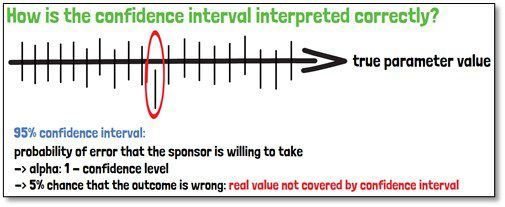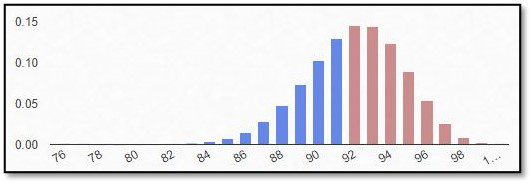# BlogBiostatistics

### What is a confidence interval?

When it is about the precision of a test result, confidence intervals are a more useful measure than p-values, which only reflect the statistical significance. Confidence intervals provide additional information about how large the effect could be, so answering the question how the clinical relevance for the finding is.Biostatistics

### What is the binominal distribution?

If an outcome variable that can be specified in two response possibilities only, data can be analyzed as binary based on a binomial distribution, where each observation of a defined number of “experiments” represents one of the two outcomes, and the probability of success is the same for each observation.
In this part of our blog series accompanying our #video #series “5 Minutes Statistics for Clinical Trials” we explain what a #binomial #distribution reflects and what are its characteristics.